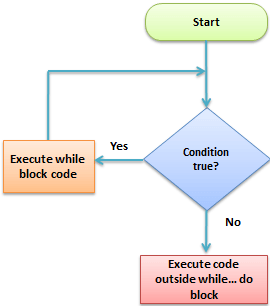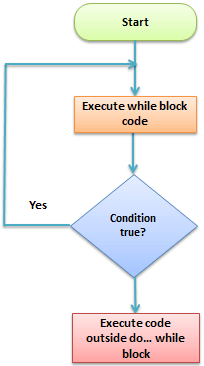# PHP While Loop and Do While Loops

Both PHP while loop and do-while loop is used to execute bulk ok code several times and using that we can execute the bulk of code several time which is inside the scope.

Most of the time the PHP while loop is used in the printing of database table values, and do-while is used if first need to print and then if condition satisfied then print in the loop.

## PHP While Loop

In case of PHP while loop the function will check the condition, if satisfied then execute the code inside of scope then again condition checking if condition returns true then the process will repeat again and again and if the condition returns false then the program will execute the code which is after the while loop.Example:

``````
<?php

\$i = 1;

while(\$i<=5){
echo "i = " . \$i;
\$i++;
}
// the php while loop will print i = 1, i = 2, i = 3, i = 4 and i = 5

echo \$i; // it will print 6
?>
``````

## PHP Do While Loop

A do-while loop is used when they’re needed the group of code to execute first whether the condition of the loop may satisfy or not, and then needed to check the condition and if the condition returns true the code will execute and the checking and execute process will be repeated until the condition returns false, and after the condition returns false the code will execute the code written after the do-while loop.PHP Do While Loop

Example:

``````
<?php

\$i = 1;

do{
echo "i = " . \$i;
\$i++;
}while(\$i<=5);
// the php while loop will print i = 1, i = 2, i = 3, i = 4 and i = 5

echo \$i; // it will print 5
?>
``````

I hope that the post PHP While Loop and Do While Loops helped you to learn PHP, and you have started to make the while and do-while loop.